FreeBuf.COM网络安全行业门户，每日发布专业的安全资讯、技术剖析。FreeBuf+小程序

Micropython进阶 | 如何用单片机制作鞋码匹配仪 金币
2018-01-10 13:00:45

## 2. 所需元器件（某宝能买到 挺便宜的）

· 超声波模块 1个

· TPYBoard v102板子 1块

· 四位数码管 1个

· micro USB数据线 1条

· 杜邦线 若干

## 3. 超声波模块工作原理

(1) 采用IO口TRIG触发测距，给最少10us的高电平信号。

(2) 模块自动发送 8 个 40khz 的方波，自动检测是否有信号返回。

(3) 有信号返回，通过 IO 口 ECHO 输出一个高电平，高电平持续的时间就是超声波从发射到返回的时间。测试距离=(高电平时间*声速(340M/S))/2。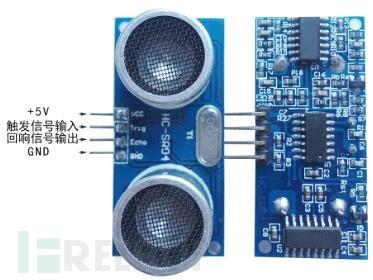## 4. 控制四位数码管显示数字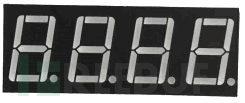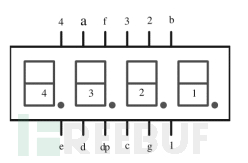（2）a、b、c、d、e、f、g、dp（h）是“段选”针脚，用法和1位数码管一致，用来显示具体的数值。

TPYBoard v10x开发板

1

X3

2

X4

3

X5

4

GND(鞋码匹配用不到四位所以该脚拉低)

TPYBoard v10x开发板

a

X6

b

X7

c

X8

d

Y9

e

Y10

f

Y11

g

Y12

dp(h)

## 5. 接线图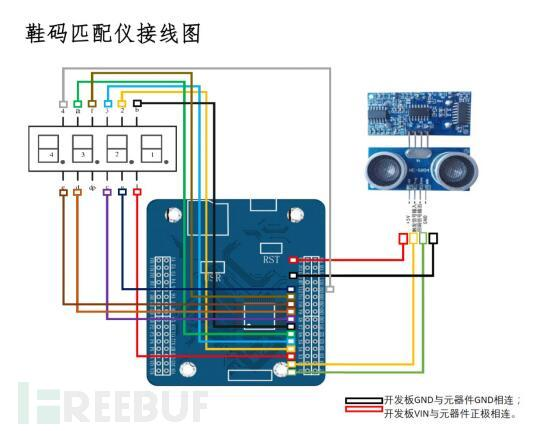## 6. 源代码

``````import pyb
from pyb import Pin
from pyb import Timer
from machine import SPI,Pin
Trig = Pin('X2',Pin.OUT_PP)
Echo = Pin('X1',Pin.IN)
num=0
flag=0
run=1
def start(t):
global flag
global num
if(flag==0):
num=0
else:
num=num+1
def stop(t):
global run
if(run==0):
run=1
start1=Timer(1,freq=10000,callback=start)
stop1=Timer(4,freq=2,callback=stop)
sizecc = [35,36,37,38,39,40,41,42,43,44,45,46,47]#280-47 -5
while True:
if(run==1):
Trig.value(1)
pyb.udelay(100)
Trig.value(0)
while(Echo.value()==0):
Trig.value(1)
pyb.udelay(100)
Trig.value(0)
flag=0
if(Echo.value()==1):
flag=1
while(Echo.value()==1):
flag=1
if(num!=0):
#print('num:',num)
distance=num/1000*34000/2
print('Distance')
print(distance,'mm')
cc = (int)((distance-215)/5)
if cc<0:
cc=0
elif cc>12:
cc=12
print(sizecc[cc],'cc')
flag=0
run=0``````

*本文作者：bodasister，转载请注明来自FreeBuf.COM

# micropython # 单片机

+ 收入我的专辑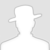• 0 文章数
• 0 评论数
• 0 关注者